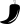# Odd Or Eveninput if types int equality comparison numbers mod

Again, the exercise comes first (with a few extras if you want the extra challenge or want to spend more time), followed by a discussion. Enjoy!

## Exercise 2 (and Solution)

Ask the user for a number. Depending on whether the number is even or odd, print out an appropriate message to the user. Hint: how does an even / odd number react differently when divided by 2?

Extras:

1. If the number is a multiple of 4, print out a different message.
2. Ask the user for two numbers: one number to check (call it `num`) and one number to divide by (`check`). If `check` divides evenly into `num`, tell that to the user. If not, print a different appropriate message.

## Discussion

Concepts for this week:

• Modular arithmetic (the modulus operator)
• Conditionals (if statements)
• Checking equality

### Modular arithmetic (the modulus operator)

We have been doing arithmetic (addition, subtraction, multiplication, division) since elementary school, and often it is useful for us to find not the answer to a division problem but the remainder when we do a division operation. This operation is called the “modulus operation.” For example, when I divide 5 by 3, the remainder is 2, and the sentence reads like this: “5 modulo 3 is 2.”

In the Python shell:

The `%` sign is exactly the modulus operator.

### Conditionals

When a computer (or a program) needs to decide something, it checks whether some condition is satisfied, which is where the term conditional comes from. Conditionals are a fancy way of saying “if statements”. If Michele was born in New York, she has an American passport. That statement is a conditional (if statement) that in this case is true. In Python this works the same way:

When the program gets to the `if` statement, it will check the value of the variable called `age` against all of the conditions, in order, and will print something to the screen accordingly. Note that `elif` is a portmanteau of “else” and “if”. So if the variable `age` holds the value 15, the statement `"can see a rated PG-13 movie"` will be printed to the screen.

Note how the statement `elif age < 17 and age > 12` has the statement `and` - you can use `or` and `not` in the same way. Understanding a bit about logic and how it works, or being able to rationally think about logic will help you get the conditions right - oh, and a lot of practice.

### Checking for equality (and comparators in general)

A fundamental thing you want to do with your program is check whether some number is equal to another. Say the user tells you how many questions they answered incorrectly on a practice exam, and depending on the number of correctly-answered questions, you can suggest a specific course of action. For integers, strings, floats, and many other variable types, this is done with a simple syntax: `==`. To explicitly check inequality, use `!=`.

Notice how in this example, the condition is redundant. In the first condition we are checking whether the variable `a` has the value 3 and in the second, we are checking whether `a` does NOT have the value 3. However, if the first condition is not true (`a` is in fact not 3), then the second condition is by definition true. So a more efficient way to write the above conditional is like this:

The same equality / inequality comparisons work for strings.